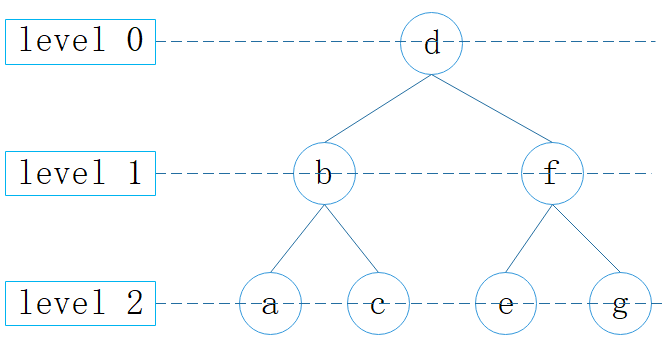# 二叉树03.一种无需队列的层序遍历算法 IDDFS

01/05 12:14

## 1. 概述

IDDFS (Iterative deepening depth-first search)：迭代加深搜索，是一种状态空间/图的搜索策略。

，相比用队列实现层序遍历所需的 $O(b^d)$ 空间复杂度，极大地节约了空间开销，b 为分支因子，d 为深度。

## 2. 使用队列实现层序遍历public void levelOrderTraversal(List<Tuple2<K, V>> kvs) {
if (root == null) {
return;
}
ArrayDeque<Node<K, V>> queue = new ArrayDeque<>(size.get() / 2);
queue.push(root);
while (!queue.isEmpty()) {
Node<K, V> p = queue.poll();
if (p.left != null) {
queue.offer(p.left);
}
if (p.right != null) {
queue.offer(p.right);
}
}
}

## 3. IDDFS

iddfs() {
// 每次循环深度+1
for (depth = 0; ; ++depth) {
// 搜索指定层
Tuple2<Boolean, Node> tuple2 = dls(depth);
// 如果指定层已无节点（表示已遍历完所有节点），退出循环
if (tuple2.getT1 == false) break;
// 如果已找到目标解，退出循环
if (tuple2.getT2 is goal) break;
}
}
​
// 搜索指定层，返回二元组：1.指定层是否有节点；2.目标解
Tuple2<Boolean, Node> dls(depth);

### 3.1. IDDFS 之递归层序遍历

IDDFS 主要用于查找目标解，而将 IDDFS 用于层序遍历，需做一个小改动：查找目标解改为遍历指定层。

public void iddfsR(List<Tuple2<K, V>> kvs) {
if (root == null) {
return;
}
int depth = 0;
while (dlsR(kvs, root, depth)) {
++depth;
}
}
​
// 遍历指定层
private boolean dlsR(List<Tuple2<K, V>> kvs, Node<K, V> node, int depth) {
// 1.是否到达指定层
if (depth == 0) {
// 1.1.depth为0，到达指定层，访问节点
// 指定层存在，总是返回 true
return true;
} else {
// 1.2 depth>0，未到指定层，继续递归
// 指定层是否存在（左子树或右子树存在指定层即返回 true）
boolean remaining = false;
// 2.1. 左子树继续递归（每次进入下一层，depth-1）
if (null != node.left && dls(kvs, node.left, depth - 1)) {
remaining = true;
}
// 2.2. 右子树继续递归（每次进入下一层，depth-1）
if (null != node.right && dls(kvs, node.right, depth - 1)) {
remaining = true;
}
// 返回指定层是否存在
return remaining;
}
}

### 3.2. IDDFS 之非递归层序遍历

public void iddfs(List<Tuple2<K, V>> kvs) {
if (root == null) {
return;
}
int depth = 0;
while (iddfs(kvs, depth)) {
++depth;
}
}
​
private boolean dls(List<Tuple2<K, V>> kvs, int depth) {
// 使用数组记录当前的分支路径
Node<K, V>[] path = new Node[depth + 1];
// 当前所在的层
int curDep = 0;
path = root;
boolean remaining = false;
while (curDep >= 0) {
Node<K, V> p = path[curDep];
if (curDep < depth) {
// 获取下层节点，用于判断是否已经遍历
Node<K, V> ch = path[curDep + 1];
// 先遍历左子树
if (p.left != null && p.left != ch) {
if (p.right != ch) {
path[++curDep] = p.left;
continue;
}
}
// 后遍历右子树
if (p.right != null && p.right != ch) {
path[++curDep] = p.right;
continue;
}
} else {
remaining = true;
}
// 已遍历左右子树（或无左右子树），回退到上层节点
--curDep;
}
return remaining;
}

PS： 之前写字典树需用到层序遍历，怕内存爆炸不敢用队列，翻了几本算法书，又在网上溜达了一圈，也没找到一个合适的算法。

### 3.3. 分析

#### 空间复杂度

IDDFS 仅需存储当前正在访问的分支路径，因此时间复杂度为 O(d)。

#### 时间复杂度

IDDFS 的时间复杂度与标准BFS 相同，即 $O(b^d)$，详细证明见参考资料1

$\sum_{d=0}^4(4+1-d)2^d=5+8+12+16+16=57$
57 小于节点数 31 的 2 倍，虽然会稍慢一些，但时间复杂度依然为 $O(b^d)$

#### 小结

IDDFS 具有与 DFS 相同的空间开销，并接近于标准 BFS 的时间效率。
IDDFS 可以很好地与其它算法相结合，有非常广泛的应用，希望更多了解可阅读参考资料1

PS：有根有据有态度的分享，如果觉得不错，记得点赞收藏哦！

## 参考资料

[ 1]   Korf R E . Depth-first iterative-deepening. 1985.### 作者的其它热门文章

0
0 收藏

0 评论
0 收藏
0